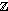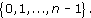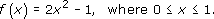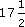The second measure of location defined in this course for a collection of data is the mean. Again, to be precise, we are discussing the sample mean, as opposed to the population mean. This is what most individuals would understand by the word ‘average’. All the items in the data set are added together, giving the sample total. This total is divided by the number of items (the sample size).

Author(s): The Open University

The data in Table 3 are the birth weights (in kg) of 50 infants suffering from severe idiopathic respiratory distress syndrome. There are two groups of infants: those who survived the condition (there were 23 of these) and those who, unfortunately, did not. The data have not been sor
Author(s): The Open UniversityWhen using a calculator many people have ‘blind
Author(s): The Open University

## Activity 21

Without using your calculator solve the following calculations.

• (a) 3 + 5 × 2 = ?

• (b) 12 − 6 + 6 = ?

• (c) 6 + (5 +
Author(s): The Open University

When calculating an answer it is important that you give careful consideration to the order of operations used in the calculation. If you are using a mixture of operations remember that certain operations take priority in a calculation. Consider the following, apparently, simple sum.

1 + 2 × 3 = ?

Did you give 7 as your response, or 9?

The correct answer is 7 but can you explain why?

If you have a calculator handy, check that it
Author(s): The Open University

After studying this course, you should be able to:

• round a given whole number to the nearest 10, 100, 1000 and so on

• round a decimal number to a given number of decimal places or significant figures

• use rounded numbers to find rough estimates for calculations

• use a calculator for decimal calculations involving +, −, × and ÷, giving the answer to a specified accuracy (e.g. decimal places or significant figures) and checking the ans
Author(s): The Open University

In the last subsection we stated that, for any integer n ≥ 2, the setn satisfies the same rules for addition modulo n as the real numbers satisfy for ordinary addition. When it comes to multiplicat
Author(s): The Open University

The Division Algorithm tells us that all the possible remainders on division by an integer n lie in the setWe denote this set by [Image_Link]https://www.open.edu
Author(s):
The Open University

In OpenLearn course Diagrams, graphs and charts you saw how a scale is used on plans of houses and other structures. The scale makes it possible to take a length on the plan and calculate the correspond
Author(s): The Open University

## Question 1

Which of these triangles are similar?

There is another kind of symmetry which is often used in designs. It can be seen, for instance, in a car wheel trim.Look at the trim on the left. It does not have line symmetry but
Author(s): The Open University

Two straight lines that do not intersect, no matter how far they are extended, are said to be parallel. Arrows are used to indicate parallel lines.Author(s): The Open University

If several angles make up a half turn, then the sum of those angles must be× 360° = 180°. Therefore, in the following diagram, α + β + γ + δ = 180°.

Author(s): The Open University

In this section we have seen a correspondence between complex numbers and points in the plane using Cartesian coordinates; the real part of the complex number is represented on the real axis (“horizontal”) and the imaginary part on the imaginary axis (“vertical”). We can also use polar coordinates (r,θ) in which case r, the modulus of a non-zero complex number z is positive and θ is an argument of z, defined only to within an additive intege
Author(s): The Open University

We have seen in Section 2.2 that the complex number system is obtained by defining arithmetic operations on the set R × R. We also know that elements of R × R can be represented as points in a plane. It seems reasonable to ask what insight can be obtained by representing complex numbers as
Author(s): The Open University

A fundamental concept in mathematics is that of a function.

Consider, for example, the function f defined byThis is an example of a real function, because it associates with a given real number
Author(s): The Open University

Our everyday experience of percentages includes percentage increases (like VAT at%, or a service charge of 15%) and percentage decreases (such as a discount of 15%).

For example, £8 plus
Author(s): The Open University

Proportion is another way of expressing notions of part and whole. You might say that the proportion of village inhabitants who are children is a quarter, or that the proportion of fruit juice in the punch is two thirds, or that the proportion of sand in the concrete is three quarters.

All these examples involve the fractions [Image_Link]https://www.open.edu/openlearn/ocw/pluginfile.php/104648/mod_oucontent/oucontent/1902/8
Author(s):
The Open University

The do–talk–record triad (DTR) is a description of what is likely to take place in collaborative mathematics classrooms. It is concerned with observable events, and with the learner rather than the teacher, though many teaching insights flow from it. Although the order of the triad suggests that it should be followed in a particular sequence, this is not necessarily the case. Sometimes talking comes before doing or recording before talking. It also takes time for a learner to move
Author(s): The Open University